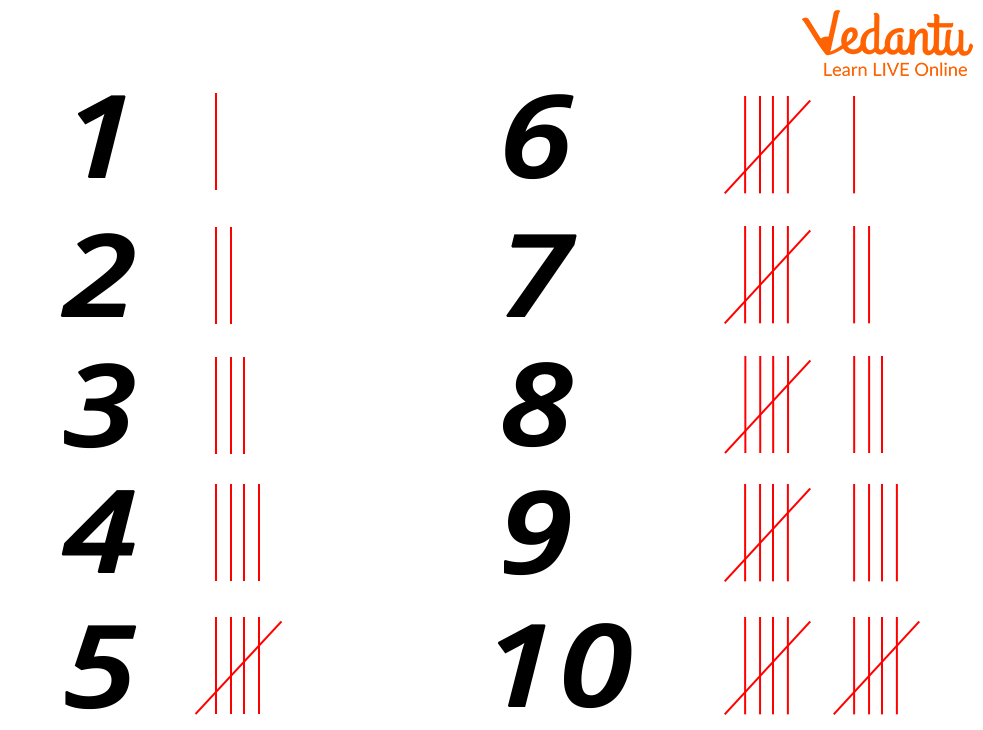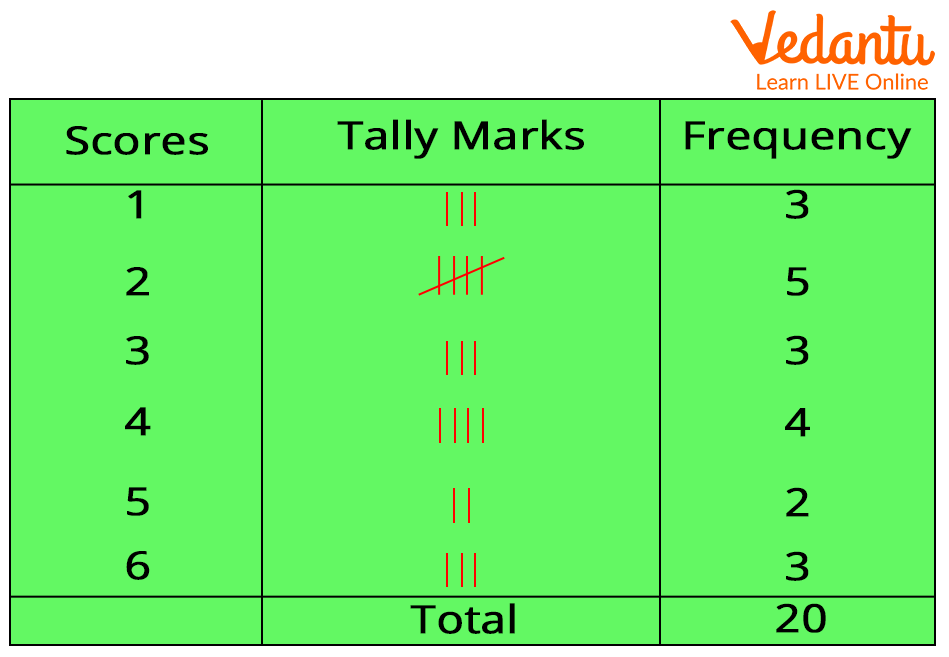Courses
Courses for Kids
Free study material
Free LIVE classes
More

# Understanding Frequency TablesLIVE
Join Vedantu’s FREE Mastercalss

## What is a Frequency?

The number of times a particular data value occurs in a given data set is referred to as its frequency. Let us understand the concept of the frequency with a simple example of grades obtained by the students of your group in the Maths project. Let’s say there are 10 students in your project group, and three of you obtained grade A in the project. Four of the students in your group get a B+, two students score a C, and one student scores D. Now, let us note down the grades obtained by each of you in a series.

A, A, A, B, B, B, B, C, C, D

Count the number of times each grade occurs in the above series. Yes, ‘A’ occurs thrice, ‘B+’ occurs four times, ‘C’ occurs twice, and ‘D’ occurs once. Now, according to the definition of frequency, we can note the frequency of each grade as follows:

Frequency of A = 3

Frequency of B = 4

Frequency of C = 2

Frequency of D = 1

Let us now learn about the frequency table.

## What is a Frequency Table?

A frequency table consists of the lists of items in a given data set and the number of times each item occurs in the data set.

Let us list the grades obtained by the students in the above example, in a frequency table.

 Grades Frequency A 3 B 4 C 2 D 1

To be more precise, here, in the above table, we have arranged the data (or grades) alongside their corresponding frequencies. The data values are listed in ascending order in frequency tables.

## What are Tally Marks?

Let us now understand tally marks in a frequency table. Tally marks make an easy way to note and record the frequencies of the values in a given data set. The frequencies 1 to 4 are represented by short straight lines, quite like the Roman 1, in tally. The below-given picture shows the tally marks for frequencies from 1 to 10.Tally marks

For frequency 1, the tally marks will be ‘I’; for frequencies 2, 3, and 4, the tally marks will be represented by repeating ‘I’ as many times as the frequency. However, the tally marks for frequency 5 is represented by oblique strike-through on the tally marks for 4.

Again, we represent frequency 6 as ‘I’ after the tally for 5. The same process is repeated to represent the rest of the frequencies in tally marks.

## How to Create a Frequency Table?

Step 1: Make a table with three columns.

Step 2: List the data in ascending order in the first column.

Step 3: The second column is for tally marks. Count the number of times each data value occurs in the given data set and write the tally marks accordingly.

Step 4: The third column is for frequencies. Count the tally marks in the second column for each data value and write the corresponding frequency in the third column.Frequency Table Example

## Solved Examples

Let us now go through a few solved examples on frequency tables.

Example 1:

The marks obtained in an English test by a class of 20 students are given below.

(Full marks: 30)

25, 23, 28, 21, 24, 23, 23, 25, 22, 21, 28, 21, 23, 25, 28, 21, 25, 24, 22, 22

Represent the above-given marks in a frequency table with tally marks.

Solution:

 Marks Tally Marks Frequency 21 IIII 4 22 III 3 23 IIII 4 24 II 2 25 IIII 4 28 III 3

Example 2:

Mary and Alice had a basketball challenge. The basket was high enough for them and each of them was given 3 chances to score. Mary’s scores were 0, 0, and 0. Alice’s scores were 1,1, and 0. Make a frequency table for the scores of Mary and Alice in the match.

 Scores Tally Marks Frequency 0 IIII 4 1 II 2

## What is Class Interval?

When the data set is spread out over a long range of values, the frequency table becomes clumsy if we make a separate row for each data. So, the data is classified into equal groups or class intervals for convenience. At most, a neat frequency table can have 5 to 10 rows, and each class interval should begin with a multiple of the group size.

For example, if the chosen group size is 10, the groups should ideally begin with 10, 20, 30, 40, 50, etc. Each group is referred to as a class interval in the frequency table.

The frequency of a class interval or group is determined by the number of data values occurring in the data set from the class interval.

## Solved Example

Make a frequency distribution table for the following data:

11, 22, 4, 9, 0, 23, 14, 17, 7, 3, 28, 13, 21, 29, 7, 21, 4, 30, 17, 26, 9

Solution:

Let us first understand the range of the given data set, 0 to 30.

To have 5 to 10 rows in the frequency table, we can choose a class interval of 5.

 Class Intervals Tally Marks Frequency 0-5 IIII 4 5-10 IIII 4 10-15 III 3 15-20 II 2 20-25 IIII 4 25-30 IIII 4

Frequency tables have applications in various fields. To strengthen the understanding of frequency and frequency tables, kids may try to count and group their toys or stationery, like pens or colour pencils, and note the numbers in a frequency table. They may also note down the scores obtained by their friends in a particular test and arrange them in a frequency distribution table. The more they apply the concept of frequency tables in their day-to-day life, the more their analytical skills will develop. Therefore, practising frequency table worksheets will help kids organise and analyse any kind of data in the long run.

Last updated date: 28th Sep 2023
Total views: 139.2k
Views today: 4.39k

## FAQs on Understanding Frequency Tables

1. What is a class interval in a frequency distribution table?

When a given data set belongs to a long-range, it is divided into groups of equal size for an ideal frequency distribution table. These groups have an equal interval and are referred to as class intervals in the frequency table.

2. How many columns are there in a frequency table?

There are generally three columns in a frequency table, for the data values, tally marks, and frequency. For advanced frequency distributions, wherein, cumulative frequency has to be calculated, the fourth column for cumulative frequency is also included in the table.